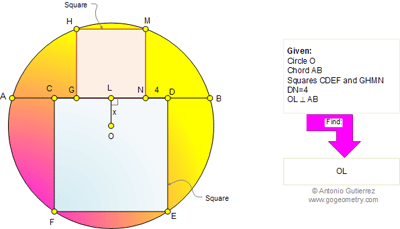## Monday, May 23, 2011

### Geometry Problem 605: Circle, Square, Chord, Perpendicular, Metric Relations

Geometry Problem
Click the figure below to see the complete problem 605.1.Let 2a, 2b be the sides of the squares (a>b)

Note a-b = 4 and

(2a-x)^2 + a^2 = r^2 = (2b+x)^2 + b^2

5(a^2-b^2)= 4(a+b)x,

Hence x = 5(a-b)/4 = 5

2.http://img836.imageshack.us/img836/732/problem605.png
Let R= radius of the circle
Note that MN=2.LN and CF=2.CL
So 3 points F, L, M are collinear
Distances LN and LC are the solutions of system equations:
1. equation y=2.t represent line FM
2. equation t^2+(y+x)^2=R^2 represent circle radius R, center O
2 positive solutions are LN= abs(t1)=1/5(-2x+SQRT(5R^2-x^2))
And LC=abs(t2)=1/5(2x+SQRT(5R^2-x^2))
DB=LC-LN=4/5.x=4

So x=5

Peter Tran